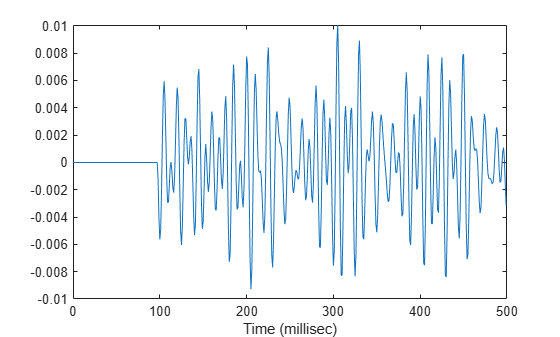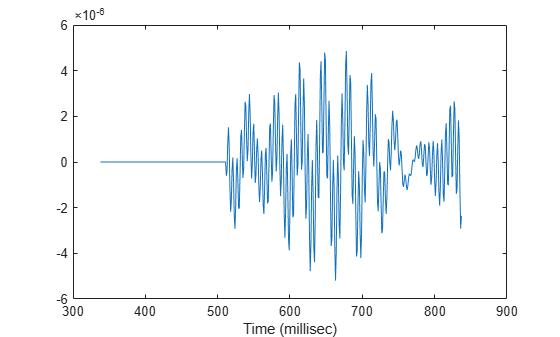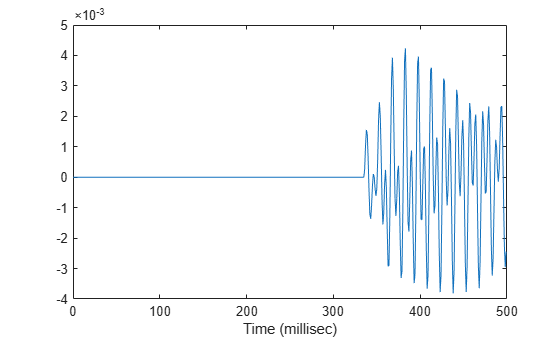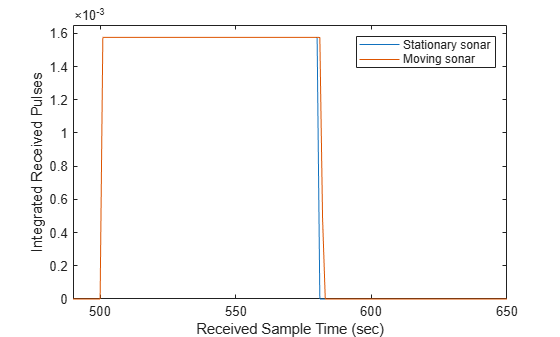# phased.MultipathChannel

Propagate signals in multipath channel

## Description

The `phased.MultipathChannel` System object™ propagates a signal through a multipath channel. To run the object, you must provide characteristics for each path: time delay, gain, Doppler factor, reflection loss, and spreading loss.

For sonar applications, you can use the `phased.IsoSpeedUnderwaterPaths` System object to generate channel path characteristics. You can also supply these characteristics independently.

To model signal propagation through a multipath channel:

1. Define and set up the propagator. You can set `phased.MultipathChannel` properties at construction time or leave them to their default values. See Construction. Some properties that you set at construction time can be changed later. These properties are tunable.

2. To compute the propagated signal, call the `step` method of `phased.MultipathChannel`. The output of the `step` method depends on the properties of the `phased.MultipathChannel` System object. You can change tunable properties at any time.

Note

Instead of using the `step` method to perform the operation defined by the System object, you can call the object with arguments, as if it were a function. For example, `y = step(obj,x)` and `y = obj(x)` perform equivalent operations.

## Construction

`propagator = phased.MultipathChannel` creates a signal propagator System object for a multipath underwater channel.

`propagator = phased.MultipathChannel(Name,Value)` creates a signal propagator System object with each specified property `Name` set to the specified `Value`. You can specify additional name and value pair arguments in any order as (`Name1,Value1`,...,`NameN,ValueN`).

## Properties

expand all

Signal carrier frequency, specified as a positive real-valued scalar. Units are in Hz.

Example: `10000`

Data Types: `double`

Signal sample rate, specified as a positive real-valued scalar. Units are in Hz. The System object uses this quantity to calculate the propagation delay in units of samples.

Example: `3e3`

Data Types: `double`

Source of the maximum delay value, specified as `'Auto'` or `'Property'`. When you set this property to `'Auto'`, the channel automatically allocates enough memory to simulate the propagation delay. When you set this property to `'Property'`, you can specify the maximum delay by using the `MaximumDelay` property. Signals arriving after the maximum delay are ignored.

Maximum signal delay, specified as a positive scalar. Delays greater than this value are ignored. Units are in seconds.

#### Dependencies

To enable this property, set the `MaximumDelaySource` property to `'Property'`.

Data Types: `double`

Interpolation method used to implement signal fractional delay and Doppler time-dilation and compression, specified as `'Linear'` or `'Oversample'`. When this property is set to `'Linear'`, the input signal is linearly interpolated directly onto a uniform grid to propagate the signal. When this property is set to `'Oversample'`, the input signal is resampled to a higher rate before linear interpolation. For broadband signals, oversampling preserves spectral shape.

Data Types: `char`

## Methods

 reset Reset state of System object step Propagate signal through multipath sound channel
Common to All System Objects
`release`

Allow System object property value changes

## Examples

collapse all

Create a five-path underwater sound channel and compute the propagation path matrix, the Doppler factor, and the absorption loss. Assume that the source is stationary and the receiver is moving along the x-axis toward the source at 20 km/h. Assume the default one-way propagation.

Create the channel and specify the source and receiver locations and velocities.

```numpaths = 5; channel = phased.IsoSpeedUnderwaterPaths('ChannelDepth',200,'BottomLoss',10, ... 'NumPathsSource','Property','NumPaths',numpaths); tstep = 1; srcpos = [0;0;-160]; rcvpos = [100;0;-50]; speed = -20*1000/3600; srcvel = [0;0;0]; rcvvel = [speed;0;0];```

Compute the path matrix, Doppler factor, and losses.

`[pathmat,dop,absloss] = channel(srcpos,rcvpos,srcvel,rcvvel,tstep);`

Create 500 samples of a 100 Hz signal. Assume all the paths have the same signal. Propagate the signals to the receiver.

```fs = 1e3; nsamp = 500; propagator = phased.MultipathChannel('OperatingFrequency',10e3,'SampleRate',fs); t = [0:(nsamp-1)]'/fs; sig0 = sin(2*pi*100*t); sig = repmat(sig0,1,numpaths); propsig = propagator(sig,pathmat,dop,absloss);```

Plot the real part of the coherent sum of the propagated signals.

```plot(t*1000,real(sum(propsig,2))) xlabel('Time (millisec)')```Create a seven-path underwater sound channel and display the propagation path matrix. Assume that the source is stationary and that the receiver is moving along the x-axis toward the source at 20 km/h. Assume two-way propagation.

```speed = -20*1000/3600; numpaths = 7; csound = 1515.0; channel = phased.IsoSpeedUnderwaterPaths('ChannelDepth',200, ... 'PropagationSpeed',csound,'BottomLoss',10,'NumPathsSource','Property', ... 'NumPaths',numpaths,'TwoWayPropagation',true); tstep = 1; srcpos = [0;0;-160]; tgtpos = [500;0;-50]; srcvel = [0;0;0]; tgtvel = [speed;0;0];```

Obtain the path matrix, Doppler factor, loss, and target reflection and transmit angles.

`[pathmat,dop,aloss,tgtangs,srcangs] = channel(srcpos,tgtpos,srcvel,tgtvel,tstep);`

Create a 100 Hz signal with 500 samples. Assume that all the paths have the same signal but with different amplitudes. Then, propagate the signals to the target and back. You can use the angle information to calculate any angular dependence of the source and target responses. Each channel can have a different amplitude. This example uses a simple cosine model.

```fs = 1e3; nsamp = 500; propagator = phased.MultipathChannel('OperatingFrequency',10e3,'SampleRate',fs); t = [0:(nsamp-1)]'/fs; ampsrc = cosd(srcangs(2,:)); amptgt = cosd(tgtangs(2,:)); sig0 = sin(2*pi*100*t); sig = repmat(sig0,1,numpaths); amptotal = ampsrc.^2.*amptgt; sig = bsxfun(@times,amptotal,sig);```

Because of the finite propagation delay, the first call to the propagator does not return the signal. Call `propagator` twice to obtain the returned signal.

```propsig = propagator(sig,pathmat,dop,aloss); propsig = propagator(sig,pathmat,dop,aloss);```

Plot the real part of the coherent sum of the propagated signals. Compute the round trip time.

```rng = rangeangle(srcpos,tgtpos); tr = rng/csound; plot((t+tr)*1000,real(sum(propsig,2))) xlabel('Time (millisec)')```Create an underwater sound channel and plot the combined received signal. Automatically find the number of paths. Assume that the source is stationary and that the receiver is moving along the x-axis toward the source at 20 km/h. Assume the default one-way propagation.

```speed = -20*1000/3600; channel = phased.IsoSpeedUnderwaterPaths('ChannelDepth',200,'BottomLoss',5, ... 'NumPathsSource','Auto','CoherenceTime',5); tstep = 1; srcpos = [0;0;-160]; rcvpos = [500;0;-50]; srcvel = [0;0;0]; rcvvel = [speed;0;0];```

Compute the path matrix, Doppler factor, and losses. The propagator outputs 51 paths output but some paths can contain `Nan` values.

`[pathmat,dop,absloss,rcvangs,srcangs] = channel(srcpos,rcvpos,srcvel,rcvvel,tstep);`

Create of a 100 Hz signal with 500 samples. Assume that all the paths have the same signal. Use a `phased.MultipathChannel` System object™ to propagate the signals to the receiver. `phased.MultipathChannel` accepts as input all paths produced by `phased.IsoSpeedUnderwaterPaths` but ignores paths that have `NaN` values.

```fs = 1e3; nsamp = 500; propagator = phased.MultipathChannel('OperatingFrequency',10e3,'SampleRate',fs); t = [0:(nsamp-1)]'/fs; sig0 = sin(2*pi*100*t); numpaths = size(pathmat,2); sig = repmat(sig0,1,numpaths); propsig = propagator(sig,pathmat,dop,absloss);```

Plot the real part of the coherent sum of the propagated signals.

```plot(t*1000,real(sum(propsig,2))) xlabel('Time (millisec)')```Compare the duration of a propagated signal from a stationary sonar to that of a moving sonar. The moving sonar has a radial velocity of 25 m/s away from the target. In each case, propagate the signal along a single path. Assume one-way propagation.

Define the sonar system parameters: maximum unambiguous range, required range resolution, operating frequency, and propagation speed.

```maxrange = 5000.0; rngres = 10.0; fc = 20.0e3; csound = 1520.0;```

Use a rectangular waveform for the transmitted signal.

```prf = csound/(2*maxrange); pulseWidth = 8*rngres/csound; pulseBW = 1/pulseWidth; fs = 80*pulseBW; waveform = phased.RectangularWaveform('PulseWidth',pulseWidth,'PRF',prf, ... 'SampleRate',fs);```

Specify the sonar positions.

```sonarplatform1 = phased.Platform('InitialPosition',[0;0;-60],'Velocity',[0;0;0]); sonarplatform2 = phased.Platform('InitialPosition',[0;0;-60],'Velocity',[0;-25;0]);```

Specify the target position.

`targetplatform = phased.Platform('InitialPosition',[0;500;-60],'Velocity',[0;0;0]);`

Define the underwater path and propagation channel objects.

```paths = phased.IsoSpeedUnderwaterPaths('ChannelDepth',100, ... 'CoherenceTime',0,'NumPathsSource','Property','NumPaths',1, ... 'PropagationSpeed',csound); propagator = phased.MultipathChannel('SampleRate',fs,'OperatingFrequency',fc);```

Create the transmitted waveform.

```wav = waveform(); nsamp = size(wav,1); rxpulses = zeros(nsamp,2); t = (0:nsamp-1)/fs;```

Transmit the signal and then receive the echo at the stationary sonar.

```[pathmat,dop,aloss,~,~] = paths(sonarplatform1.InitialPosition, ... targetplatform.InitialPosition,sonarplatform1.InitialVelocity, ... targetplatform.InitialVelocity,1/prf); rxpulses(:,1) = propagator(wav,pathmat,dop,aloss);```

Transmit and receive at the moving sonar.

```[pathmat,dop,aloss,~,~] = paths(sonarplatform2.InitialPosition, ... targetplatform.InitialPosition,sonarplatform2.Velocity, ... targetplatform.Velocity,1/prf); rxpulses(:,2) = propagator(wav,pathmat,dop,aloss);```

Plot the received pulses.

```plot(abs(rxpulses)) xlim([490 650]) ylim([0 1.65e-3]) legend('Stationary sonar','Moving sonar') xlabel('Received Sample Time (sec)') ylabel('Integrated Received Pulses')```The signal received at the moving sonar has increased in duration compared to the stationary sonar.

 Urick, R.J. Principles of Underwater Sound, 3rd Edition. New York: Peninsula Publishing, 1996.

 Sherman, C.S. and J. Butler Transducers and Arrays for Underwater Sound. New York: Springer, 2007.

 Allen, J.B. and D. Berkely, “Image method for efficiently simulating small-room acoustics”, J. Acoust. Soc. Am, Vol 65, No. 4. April 1979.